# How do I Insert a Checkmark in Excel 2010-2016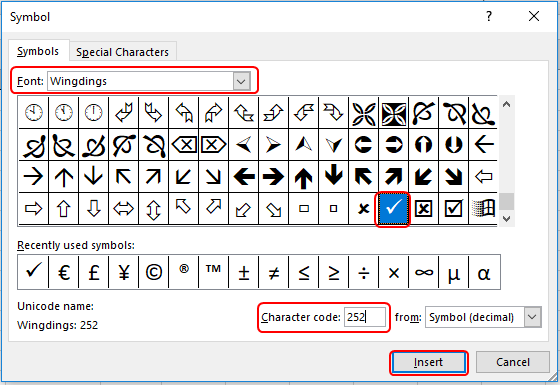In this article, we will learn How do I Insert a Checkmark in Excel 2010-2016.

What is a checkmark and where do we use it ?

Checkmark is a tick symbol used in Wingdings format. While writing some information or making a checklist, where elements are marked using a small tick mark. All the elements which are considered are marked with these tick marks. Many of us like to use the same in Excel. It makes data presentable and easy to understand.

Example :

All of these might be confusing to understand. Let's understand how to use the function using an example. Here First is by changing font style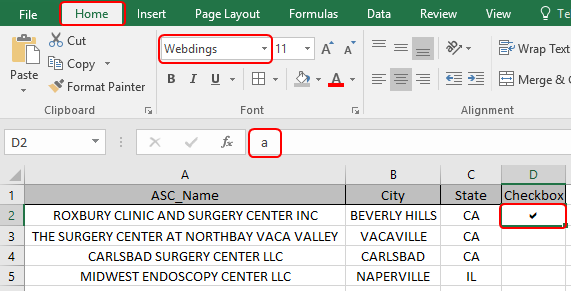Go to Home > Select webdings in font style option and type alphabet a from the keyboard. You will see a checkmark on the selected cell.

The second method is by adding checkmark from symbols option

Go to Insert > Symbol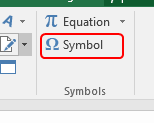Symbol dialog box appears on your sheet.Select Wingdings in Font and type character code 252. Insert Checkmark.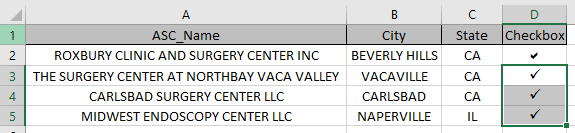As you can see checkmarks are added.

Check mark option is enabled in the format cell option. Use the Ctrl + 1 on the cell and select font option and then select wingdings 2. Wingdings 2 operate capital P as check mark in excel.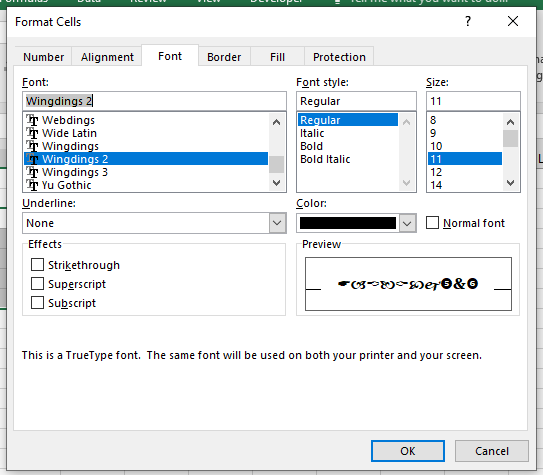IF function excel tests the condition and returns value either it's True or False.

Syntax of IF function:

 = IF ( Logic_test , [value_if_true] , [Value_if_false] )

Logic test : operation to perform

The COUNTIF function of excel just counts the number of cells with a specific condition in a given range.

Syntax Of COUNTIF Statement

 = COUNTIF ( range , condition )

Range: it is simply the range in which you want to count values.

Condition: This is where we tell Excel what to count. It can be a specific Text (should be in “”), a number, a logical operator(=,><,>=,<=,<>) and wild card operators (*,?).

We will construct a formula to do our task. In logic_test argument we will use the countif function and for the value_if_True use the Capital P.

 = IF ( COUNTIF ( array , cell_value ), "P" , "" )

Here we have a list of colors data and and a list of colors which needed to be checked with colors data.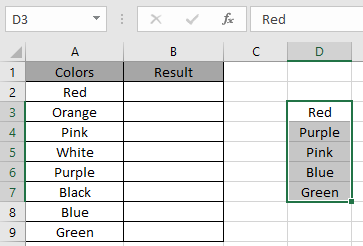Here we need to use the formula to get the check mark symbol wherever required.

So we will use the formula to get the checkmark

 = IF ( COUNTIF ( D3:D7 , A2 ), "P" , "" )

Explanation:

COUNTIF will return 1 if the value is found or returns 0 if not. So the COUNTIF function works fine logic test for the IF function.

The IF function returns capital P if the value is 1 or else it returns an empty string if the value is 0.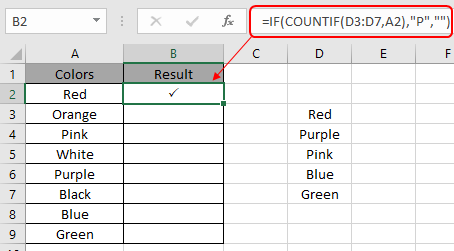Here the arguments to the function are given as cell reference.

Copy the formula to another cell using the Ctrl + D shortcut or drag down option in excel.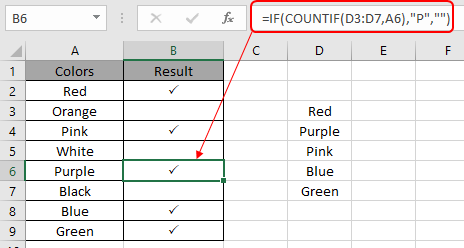As you can see the check marks wherever required.

Notes:

1. The function returns an error if a non-numeric value is used without a quote sign ("value").
2. The function returns the check mark using the IF function with COUNTIF function.

Hope this article about How do I Insert a Checkmark in Excel 2016 is explanatory. Find more articles on calculating values and related Excel formulas here. If you liked our blogs, share it with your friends on Facebook. And also you can follow us on Twitter and Facebook. We would love to hear from you, do let us know how we can improve, complement or innovate our work and make it better for you. Write to us at info@exceltip.com.

Related Articles:

How to use the Shortcut To Toggle Between Absolute and Relative References in Excel : F4 shortcut to convert absolute to relative reference and same shortcut use for vice versa in Excel.

How to use Shortcut Keys for Merge and Center in Excel : Use Alt and then follow h, m and c to Merge and centre cells in Excel.

How to Select Entire Column and Row Using Keyboard Shortcuts in Excel : Use Ctrl + Space to select whole column and  Shift + Space to select whole row using keyboard shortcut in Excel

Paste Special Shortcut in Mac and Windows : In windows, the keyboard shortcut for paste special is Ctrl + Alt + V. Whereas in Mac, use Ctrl + COMMAND + V key combination to open the paste special dialog in Excel.

How to Insert Row Shortcut in Excel : Use Ctrl + Shift + = to open the Insert dialog box where you can insert row, column or cells in Excel.

Popular Articles :

How to use the IF Function in Excel : The IF statement in Excel checks the condition and returns a specific value if the condition is TRUE or returns another specific value if FALSE.

How to use the VLOOKUP Function in Excel : This is one of the most used and popular functions of excel that is used to lookup value from different ranges and sheets.

How to Use SUMIF Function in Excel : This is another dashboard essential function. This helps you sum up values on specific conditions.

How to use the COUNTIF Function in Excel : Count values with conditions using this amazing function. You don't need to filter your data to count specific values. Countif function is essential to prepare your dashboard.

1.